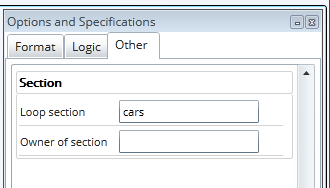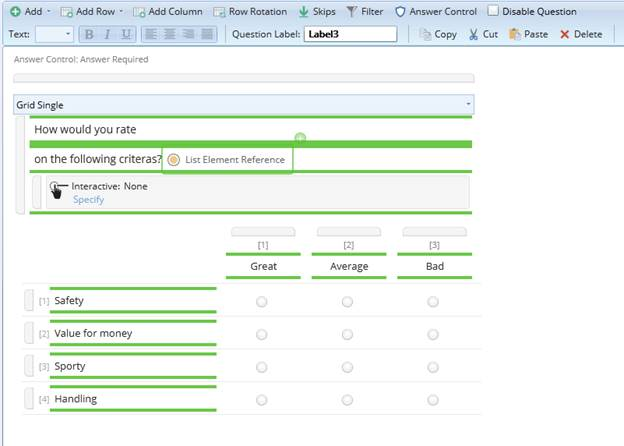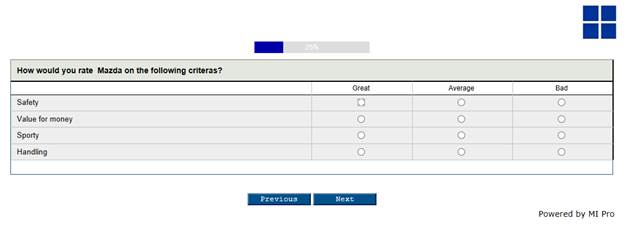# How to repeat groups of questions (looping)

In many questionnaires, there may be a set of questions that are going to be asked repeatedly. These questions are known as “loops”. Loops are based either on a question about how many times the same set of questions will be asked (for example, in a household questionnaire based on the number of household members, you might want to ask the same set of questions such as gender, age, and position for each household member) or against a list of similar items (for example, a brand list, where a set of questions is asked about each brand).

Generally, you need to make the same set of questions and place them in a section. The section is then copied the required number of times. All IDs and logic within the section must be updated. If you need to add a new question to the set or change something, you need to make change in all occurrences.

As with lists, Research Studio allows you to define the questions in one place and then easily refer to the questions/section by looping a fixed (max) number of times or by specifying the list of brands to loop over. The set of questions is defined as a “macro” and then copied or duplicated the required number of times by the publishing system.

When using a list to create a loop, you may use the “List element reference” element anywhere that text can go in a “text area”. For example, the same list of cars as used in the previous chapter (Reusing the same list or scale in a questionnaire), ‘Mazda’, ‘Toyota, and ‘Ford’ can be reused when creating rows in a question asking “What do you think about?” First time around the loop, the “List element reference” will be replaced by ‘Mazda, second time by ‘Toyota’, and third time by ‘Ford’.

Looping – an example

Step 1 - To create a list that can be used in a loop

In this example we will use the list in an example of a knowledge question: “Which car brands do you know”

Step 2 – To reuse a list item in a loop for a particular question

1. Create a new section
• In the options menu, specify the list name that the section should loop over2. Create a question, e.g. a grid question
3. Insert a “List element reference” in the text placeholder.4. In the filter of this question, specify the following filter: \knowledge.a=\$cnt; This will perform the following
• If the respondent has answered Mazda (=1) in the knowledge question, this filter will be true for the loop number 1, Toyota (=2) for loop 2, etc. The \$cnt; will replace with the correct code for each loop
5. The same syntax, \$cnt; should also be included in the question label
• In this case Question label = Rate\$cnt; Question has been
6. Later, e.g. in the analysis, this question label will be expanded so that
• Question of Mazda will have label Rate1
• Question of Toyota will have label Rate2
• Question of Ford will have label Rate3
7. In the interview, the question Rate\$cnt; will look like this (for those having ticked Mazda)